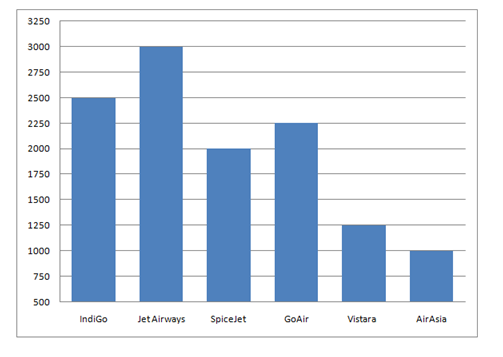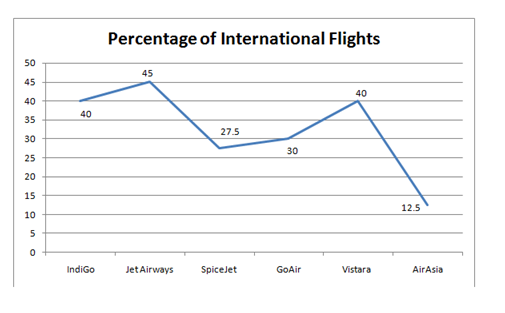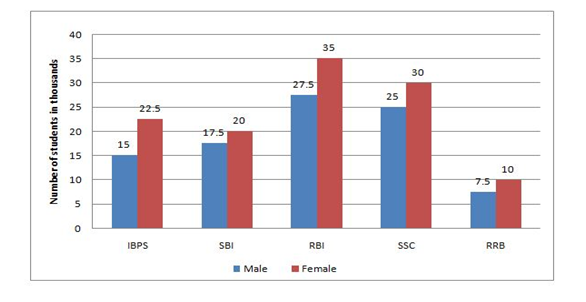# Crack SBI Clerk Prelims 2018 Sectional Full Test (Quantitative Aptitude) Day-13

Dear Readers, SBI Clerk (Junior Associates) 2018 Preliminary Examination is scheduled to be held on 23rd, 24th, and 30th of June 2018. In that case, aspirants need to speed up the preparation as there are only few days more.

To boost up your exam preparation, Our IBPS Guide team is providing a full length Sectional Tests for English, Quantitative Aptitude and Reasoning with detailed solutions to score more marks in the prelims exam. Make use of this opportunity and recommend to your friends to achieve a successful career in Banking.

You can also practice using our SBI Clerk Test Series pack prepared by our experienced professionals as per the latest exam pattern. We wish you a great success in all forthcoming exams. Click Here for SBI Clerk Practice Mock Test

#### Minimum Cut Off for this Quantitative Aptitude Section Test is: 20

[WpProQuiz 2602]

Click “Start Quiz” to attend these Questions and view Solutions

Direction (Q. 1-5): Study the graphs carefully to answer the questions that follow: Total number of flights in 6 different airlines and the percentage of International Flights in them1. The total number of flights in Spice Jet, is approximately what percent of the number of National Flights in Vistara and number of International Flights in Jet Airways together?
1. 95%
2. 90%
3. 91%
4. 87%
5. 85%
1. What is the average number of National Flights in IndiGo and Jet Airways and International Flights in Vistara and AirAsia together?
1. 1025
2. 943.75
3. 972.50
4. 912.25
5. 873.75
1. What is the respective ratio of the number of International Flights in SpiceJet and GoAir together to the number of National Flights in Vistara and AirAsia together?
1. 48 : 77
2. 39 : 56
3. 49 : 65
4. 7 : 13
5. 65 : 49
1. What is the total percentage of National Flights in Spice Jet and Air Asia together from the total number of Flights of Spice Jet and Air Asia (rounded off to two digits after decimal)
1. 71.70
2. 70.50
3. 73.60
4. 77.50
5. 74.90
1. What is the total number of National Flights in IndiGo, GoAir and Vistara together?
1. 4095
2. 3755
3. 3975
4. 3645
5. 3825

Directions (Q. 6-10) : What value will come in place of question mark (?) in the given questions ? (You are not expected to calculate the exact value)

6). 18.05 x 8.99 – 52.02 =?

1. 140
2. 75
3. 160
4. 110
5. 150

7). 149.96 x 3.02 – 114.57 x 1.93 =?

1. 170
2. 220
3. 310
4. 190
5. 260

8). 45.04 ÷ 4.97 = ? ÷ 12.99

1. 104
2. 91
3. 120
4. 117
5. 143

9). 135.59 ÷ 7.62 x 2.93 = 75.01% of?

1. 80
2. 60
3. 40
4. 20
5. 68

10). 75.05% of (289.96 + 142.01) = ?2

1. 10
2. 16
3. 14
4. 18
5. 12
1. Two pipes M and N can fill a cistern in 24 min and 32 min respectively. If both pipes are opened together, then after how many minutes N should be closed so that the tank is full in 18 minutes?
1. 6
2. 10
3. 8
4. 21
5. None of these
1. Since price of the Banana goes down by 20% a man can now buy 2 bananas more in 40 Rs. What was the original price of one banana?
1. 25
2. 24
3. 23.5
4. 26
5. None of these
1. A man driving his bike at 24kmph reaches his office 5 minutes late. Had he driven 25% faster on an average he would have reached 4 minutes earlier than the scheduled time. How far is his office?
1. 24km
2. 72km
3. 18km
4. 56km
5. None of these
1. 40% of the employees in a factory are workers. All the remaining employees are executives. The annual income of each worker is 390rs. The annual income of each executive is Rs. 420. What is the average annual income of all the employees in the factory together?
1. 390
2. 405
3. 408
4. 415
5. None of these
1. Raghu’s monthly income is (5/4) times that of Raju’s income, where as his monthly expenses are twice that of Raju’s expenses. If each of them saves Rs 12000, then what is the annual income of Raju?
1. Rs 144000
2. Rs 192000
3. Rs 240000
4. Cannot be determined
5. Rs 234000
1. There is a rectangular room whose length, breadth and height are 12m, 10m and 4m respectively. What will be the cost of painting the four walls of the room if the rate of painting is Rs.12.60 per square metre?
1. Rs.2258.40
2. Rs.2261.90
3. Rs.2271.80
4. Rs.2208.30
5. Rs.2217.60
1. In a bag there are 6 blue ball pens and 7 red ball pens. Four ball pens are picked at random. What is the probability that two ball pens are blue and two ball pens are red?
1. 63/143
2. 76/143
3. 23/143
4. 65/140
5. 43/143
1. 15 men can finish a piece of work in 60 days. 12 men started working and in 45 days they finished a certain part of the work. If it is required to finish the remaining work in 8 days, how many men must be added to the existing workforce?
1. 33
2. 44
3. 38
4. 48
5. 36
1. A certain sum of money amounted to Rs 7200 at 5% in a time in which Rs 4800 amounted to Rs 6336 at rate of 8%. What is the sum in this case considering simple interest rate?
1. Rs 6500
2. Rs 6000
3. Rs 5600
4. Rs 6200
5. None of These
1. The incomes of A and B are in the ratio 9:4 and their expenditures are in the ratio 7:3. If savings of A and B are Rs 4000 and Rs 2500 respectively then what are the incomes of A and B respectively?
1. Rs 45000, Rs 22000
2. Rs 49500, Rs 22000
3. Rs 52500, Rs 25000
4. Rs 47500, Rs 24500
5. None of these

Directions (Q. 21-25): Study the following graph carefully to answer these questions

Number of students (Males & Females) in thousands passed out from various exams in a year.1. What is the difference between the total number of students passing out from IBPS Exam and the total number of students passing out from RRB Exam?
1. 20,500
2. 21,000
3. 10,500
4. 10,000
5. None of these
1. The number of Males passing out from IBPS Exam and SBI Exam together is what percent of the number of females passing out from RBI Exam and SSC Exam together?
1. 45
2. 40
3. 35
4. 50
5. None of these
1. The number of Females passed out from RBI Exam is approximately what percent the total number of Females passed out from all the exams together?
1. 28
2. 30
3. 36
4. 25
5. 40
1. What is the average number of students (Males & Females) passed out from all the exams together?
1. 38000
2. 48000
3. 42000
4. 51000
5. None of these
1. What is the respective ratio of the total number of Males to the total number of Females passed out from all the exams together?
1. 37:47
2. 18:25
3. 23:19
4. 25:18
5. None of these

Directions (Q 26-30): Find the missing number in the following series

26). 21, 35, 30, 44, 39, ?

1. 35
2. 43
3. 53
4. 52
5. 59

27). 8, 4.5, 5.5, 13, 56, ?

1. 448
2. 446
3. 356
4. 456
5. 454

28). 19, 16, 44, 101, ?

1. 125
2. 185
3. 165
4. 145
5. 195

29). 19, 25, 42, 71, 113, ?

1. 121
2. 144
3. 196
4. 125
5. 169

30). 42, 51, 67, 97, 148, ?

1. 227
2. 221
3. 225
4. 212
5. 217

Directions (31-35): For the two given equations I and II.

a) If p is greater than q.

b) If p is smaller than q.

c) If p is equal to q.

d) If p is either equal to or greater than q.

e) If p is either equal to or smaller than q.

31) I. 6p2+5p+1=0

II. 20q2+9q=-1

32)  I. 3p2+2p-1=0

II. 2q2+7q+6=0

33)  I. 3p2+15p=-18

II. q2+7q+12=0

34) I. p=√4/√9

II. 9q2-12q+4=0

35) I. p2+13p+42=0

II. q2=36

Direction (1-5)

Total number of flights in SpiceJet = 2000

Number of National Flights in Vistara = 1250 × 60/100 = 750

Number of International Flights in Jet Airways = 3000 × 45/100 = 1350

Total = 750 + 1350 = 2100

Required percentage

= 2000/2100 × 100

= 95%

Number of National Flights in IndiGo = 2500 × 60/100 = 1500

Number of National Flights in Jet Airways = 3000 × 55/100 = 1650

Number of International Flights in Vistara= 1250 × 40/100 = 500

Number of International Flights in AirAsia= 1000 × 12.5/100 = 125

Required average = 1/4 × (1500 + 1650 + 500 + 125)

= 1/4 × 3775

= 943.75

Number of International Flights in SpiceJet = 2000 × 27.5/100 = 550

Number of International Flights in GoAir = 2250 × 30/100 = 675

Total = 1225

Number of National Flights in Vistara = 1250 × 60/100 = 750

Number of National Flights in AirAsia = 1000 × 87.5/100 = 875

Total = 1625

Required ratio = 1225: 1625

= 49: 65

Number of National Flights in Spice Jet = 2000 × 72.5/100

= 1450

Number of National Flights in AirAsia = 1000 × 87.5/100

= 875

Total = (1450 + 875) = 2325

Required percentage = 2325/3000 ×100

= 77.5

Number of National Flights in IndiGo = 2500 × 60/100

= 1500

Number of National Flights in GoAir = 2250 × 70/100

= 1575

Number of National Flights in Vistara = 1250 × 60/100

= 750

Total = 1500 + 1575 + 750

= 3825

Direction (6-10)

18.05 x 8.99 – 52.02 =?

18 x9 – 52 =?

162 -52 =?

? = 110

149.96 x 3.02 – 114.57 x 1.93 = ?

150 x3 -115 x2 = ?

450 – 230 = ?

? = 220

45.04 ÷ 4.97 = ? ÷ 12.99

45 /5 = ?/13

9 x 13 = ?

? = 117

135.594 ÷ 7.62 x 2.93 = 75.01% of ?

(136/8) x 3 = (75/100) × ?

17 x3 = (3/4) x ?

? = 68

75.05% of (289.96 + 142.01) = ?2

75/100 x (290 + 142 ) = ?2

3/4 x432 = ?2

3 x 108 = ?2

324 = ?2

? = √324

? =18

Consider M & N pipes opened together for x min

M——24min         total work

N——-32min             96 units

Efficiency of M is 4

Efficiency of N is 3

7x+4(18-x) = 96

X = 8 minutes

2 Bananas 40 Rs

1 banana 20 Rs

(80/100)X = 20

X= 25

Original price for 1 banana = Rs. 25

Let x km be the distance

Time1 = x/24 hrs

While travelling at 25% faster speed

24 + 25% 24 = 30 kmph

Time2= x/30 hrs

Total time difference 9 minutes

x/24 – x/30= 9

x = 18km

Let total No. of employees be 100

(40 x 390 + 60 x 420)/100 = 408

Income ratio of Raghu and Raju =5:4

Expense ratio of Raghu and Raju = 2:1

Given,

Raghu’s savings = 5x-2y=12000 — (1)

Raju’s savings = 4x-y=12000 — (2)

Simplify the above equation (1) and (2), we get x= 4000

Raju’s annual income = 16000*12=Rs.192000

Area of 4 walls of a room

= 2(Length + Breadth) * Height

= 2(12 + 10)* 4 = 176m2

Required cost = 176 x 12.6 = Rs.2217.60

Total number of ball pens = n(S)

= 6 + 7 = 13

Required probability (6C2 x7C2)/ 13C4= (15x 21)/715 = 63/143

Total work = 15 x 60 = 900 units

Remaining work = 900 – 12 x 45 = 360 units

Let x men be added to finish the remaining work in 8 days

Then, (12 + x) 8 = 360

Or, 8x = 360 – 96

x= 264/8 =33

Let’s assume that the required time = T years

Hence in 2nd case, (6336 – 4800) = (4800’8’T)/100

=> T = (1536’100)/(4800’8)

=> T = 4 years

Let’s suppose that the principal amount be Rs P in 1St case.

Hence, P + (P'((5’4)/100)) = 7200

=> 1.2P = 7200

=> P = Rs 6000

Let the income of A and B be Rs 9n and 4n respectively and the expenditure of A and B be Rs 7m and 3m respectively.

Hence according to the question; 9n – 7m = 4000… (1)

Similarly; 4n – 3m = 2500… (2)

Solving (1) and (2) we will get; n = 5500, m = 6500

Hence income of A = 9n = Rs 49500, Income of B = 4n = Rs 22000

Direction (21-25)

Passed students from IBPS Exam= 37500

Passed students from RRB Exam = 17500

Difference = 37500 – 17500

= 20000

Passed Boys from IBPS Exam and SBI Exam

= 15000 + 17500

Passed Girls from RBI Exam and SSC Exam

= 35000 + 30000

% = 32500/65000 × 100 = 50%

Passed Girls from RBI Exam = 35000

Passed Girls from All exams = 117500

Approximate % = 35000 / 117500 × 100 = 29.78% = 30%

Average number of passed students in all Exams

Boys + Girls = Students

IBPS =15000 + 22500= 37500

SBI = 17500 + 20000= 37500

RBI = 27500 + 35000= 62500

SSC = 25000 + 30000= 55000

RRB = 10000 + 7500 = 17500

Total = 210000

Average = 210000/5

= 42000

Ratio between passed all boys and all girls in all exams

= Boys: Girls

= 92500: 117500

= 37: 47

Direction (26-30)

+14, -5, +14, -5 alternatively

×0.5+.05,  ×1+1,  ×2+2,   ×4+4,   ×8+8

Difference of difference

-3…………28………..57………..84

……31………….29………27

Difference of difference

6…………17………..29………..42………..56

……11………….12………13………..14

The difference of difference is 7, 14, 21, 28…

Direction (31-35)

I. 6p2+5p+1=0

(3p+1)(2p+1)=0

p=-1/3,-1/2

II. 20q2+9q+1=0

(4q+1)(5q+1)=0

q=-1/4,-1/5

∴p<q

I. 3p2+2p-1=0

(3p-1)(p+1)=0

p=1/3,-1

II. 2q2+7q+6=0

(2q+3)(q+2)=0

q=-3/2,-2

p>q

I. 3p2+15p+18=0

(3p+6)(p+3)=0

p=-2,-3

II. q2+7q+12=0

(q+4)(q+3)=0

q=-3,-4

∴p≥q

1. p=√4/√9=2/3
2. 9q2-12q+4=0

(3q-2)2=0

q=2/3

∴p=q

I. p2+13p+42=0

(p+7)(p+6)=0

p=-6,-7

II. q2=36

q=±6

∴p≤q